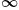Mathematics
Easy

Question

# Find the range of the function p(x) = 2 |x - 5|

## The correct answer is: [0 ,)

### To find the range of the function p(x) = 2 |x - 5|.Absolute values cannot be equal to negative.So, the range of the function p(x) = 2 |x - 5| is [0 , ∞).

Hence, the range of the function p(x) = 2 |x - 5| is  [0 , ∞).

### Related Questions to study#### With Turito Foundation.#### Get an Expert Advice From Turito.